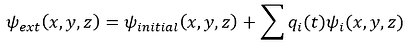# Micromachined Energy Harvesting Device for EM Application

At present, harvesting vibration energy from the environment is an attractive alternative power source for low power wireless sensing applications. Our example on the non-linear model provides insights about the hybrid frequency broadening (HFB) by capturing the energy due to different modes of vibration through a fixed-fixed beam.

The model indicated in Fig 1 indicates a schematic model of the proposed hybrid frequency broadening (HFB) mechanism for EM energy harvesting. Instead of using a common free-end cantilever beam, the design contains a pair of thin fixed-fixed beams and a large mass frame connected through the middle beam joints. The mass frame is integrated with a reflective surface in the center for monitoring its vibration behavior via the light reflection approach. We realize the design reported in the article titled “Ultra-wide frequency broadening mechanism for micro-scale electromagnetic energy harvester”, Appl. Phys. Lett. 104, 053901 (2014); https://doi.org/10.1063/1.4863565

Fig 1: Finite Element Model

In general, the displacement at any nodes can be represented as in mode decomposition:

Where the qi (t) can be derived from macromodel formula as

In the above equation, U is the model strain energy function. In general, U can be described as

We perform reduced ordered modeling simulation through the System Model Extraction (SME). It is a means by which a full three-dimensional meshed numerical model of a multi-conductor electromechanical device can be converted into a reduced-order analytical macromodel that can be inserted as a black-box element into a mixed-signal circuit simulator. This process is based on the energy method approach. We construct analytical models for each of the energy domains of the system and determine all forces as gradients of the energy.

The energy method has the advantage of making this process modular, enabling us to incorporate other energy domains into our models in the future. In addition, our energy methods are guaranteed to be energy conserving, because each stored energy shall be constructed as an analytical function, and all forces shall be computed directly from analytically computed gradients. The advantage of using SME is that the user shall construct the model and all necessary parameters of the model are considered during the simulation. Above all, this process has the ultimate benefit of constructing computationally efficient models, allowing their use in a dynamical simulator.

Initially, we reduce the degrees of freedom of the system. Rather than allow each node in a finite element model to be free to move in any direction, we constrain the motion of the system to a linear superposition of a selected set of deformation shapes. This set will act as our basic set of motion. The positional state of the system will hence be reduced to a set of generalized coordinates and each coordinate being the scaling factor by which its corresponding basis shape will contribute. Next, we construct an analytical macromodels of each of the energy domains of the system. In the case of conservative capacitive electromechanical systems, these consist of the electrostatic and kinetic energy domains. These macromodels will be the analytical functions of the generalized coordinates. (As we will see in Section Using Mode Shapes as a Basis Set, some of these energy domains will be determined as a by-product of modal analysis, avoiding the need for explicit calculation). We can then use Lagrangian mechanics to construct the equations of motion of the system in terms of its generalized coordinates. Finally, we can translate these equations of motion into an Analog hardware description language (HDL), thereby constructing a black-box model of the electromechanical system that can be inserted into an Analog circuit simulator.

Fig 3: Defining strain matrixSome of the key equations used for the conversion process are discussed in this section. In general, the deformation state and dynamics of a mechanical system can be accurately described as the linear combination of mode shape function or modal superposition.Fig 4: Local slope coordinate

Where the term on Left-hand side in the above equation represents the deformed state of the structure, the first term on the right-hand side represents the initial equilibrium state (derived from the residual stress without external loads), the second term on the right-hand side represent the displacement vector for the ith mode, qi represents the coefficients for the ith mode, which is referred to as scaling factor for mode i. The modal superposition based reduced-order modeling method is to solve each equation.Fig 5 : Displacement

Fig 7: Potential Generated

Where mi is the ith mode generalized mass, (Zeta)i is the linear modal damping ratio,  (omega)i is the ith eigenfrequency, (Zai)i is the ith model shape function (displacement vector for the ith mode). The last term on the self hand side is the sum over all the nodes of the external node force weighted by the mode shape. Um is the strain energy and Ue is the electrostatic energy. However, we do not find any relevance of electrostatic in this case.

The modal superposition based reduced-order modeling procedure includes the following steps:

• Find out “Modal Contribution”. In this step, perform the standard electromechanical relaxation analysis and solve the initial deformed state (derived from the residual stress without external loads) and the final deformed state (with mechanical loads and applied voltage). Then use the QR factorization algorithm to determine the mode contribution for the deformed state.

• Calculate the relationship of “Strain Energy vs modal amplitudes”. In this step, calculate the selected mode “Strain Energy vs modal amplitudes”.Fig 3 : Non-Linear Macromodel Element

Fig 5 : Displacement Vs frequency up-sweep at accelerations of 0.1g, 0.2g, 0.4g,

0.6g and 1.0g

Fig4: Output voltage against frequency up-sweep at accelerations 0.2g, 0.4g (Experimental)